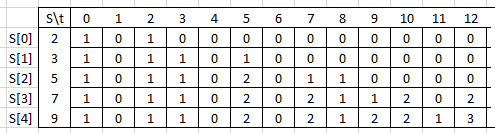# Question & Answer: St 0 1 2 3 45 6 7 8 9 10 11 12 S[02 10 10 00 0 0 0 0 0 00 S[1 31 0 11 01 0 0 0 0 0 00 S[251 0 11 02 0 1 1 0 0 00 S[371 0 1 1 02 0…..

I have written a dynamic programming algorithm that finds the total amount of subsets that sum up to a target value. However, I am having trouble developing a function to recover the solution (that is, print out the actual subsets).

For example, let’s take the set [2,3,5,7,9] with the target 12. My algorithm calculates that there are 3 subsets. The output table is shown in the image below.But, with more complicated solutions, how would I use my output table to recursively recover a solution? Any assistance is appreciated.

St 0 1 2 3 45 6 7 8 9 10 11 12 S[02 10 10 00 0 0 0 0 0 00 S[1 31 0 11 01 0 0 0 0 0 00 S[251 0 11 02 0 1 1 0 0 00 S[371 0 1 1 02 0 2112 02 S49 10 11 02 0 2 12 2 13

Without your dynamic programming algorithm, it is difficult to analyze and answer.

However, I derived a method that works for all possible cases

Algorithm Sketch:

Iterate through the results of the target column in ascending order.

When the result increments, you have identified another subset and have found the largest value in that subset.

To find the remaining values in the subset, “make change” the way a store clerk would. In other words, walk back down the set values, subtracting from the remaining total as you can.

Sample Run:

For your given example of target sum = 12 for subsets within set [2,3,5,7,9]:

resultCount = 0

result(12,2) is 0; this result – resultCount = 0, so no new subsets

result(12,3) is 0; this result – resultCount = 0, so no new subsets

result(12,5) is 0; this result – resultCount = 0, so no new subsets

result(12,7) is 2; this result – resultCount = 1, so resultCount = 2

and new subset = 

and remaining = 12 – 7 = 5

5 = 5, so subset = [7,5] and remaining = 0 (subset complete)

result(12,7) is 2; this result – resultCount = 1, so resultCount = 2

and new subset = 

and remaining = 12 – 7 = 5

Again the algorithm is called internally for second time.

5=3+2, so subset=[7,3,2] and remaining = 0 (subset complete)

result(12,9) is 3; this result – resultCount = 1, so resultCount = 3

and new subset = 

and remaining = 12 – 9 = 3

7 > 3, so remaining is still 4

5 > 3, so remaining is still 4

3 = 3, so subset = [9,3] and remaining = 3 – 3 = 0(subset complete)

End of run with 3 subsets identified:

[7,5] , [7,3,2], [9,3]

Note: Based on your output table, there would be 3 subsets.